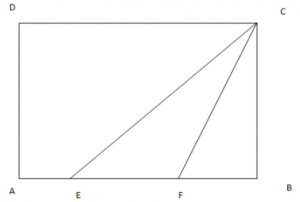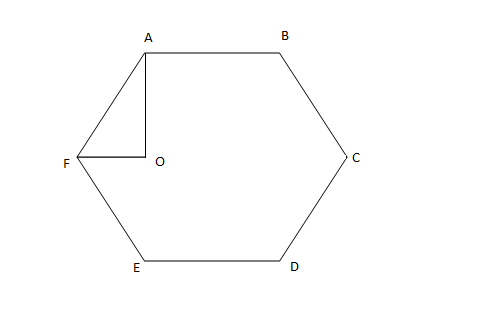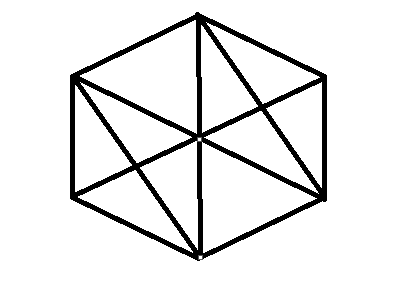# Mensuration Questions for CAT

1
2880

Mensuration is the part of Geometry. Basically Mensuration deals with the shapes (Volumes, lengths and areas). The basic figures like cuboid, cube, cone, sphere, pyramid, (hexagonal/circular) properties need to be remembered to solve questions on mensuration for CAT. We have give some mensuration questions based on previous CAT papers.

## Mensuration Questions for CAT with answers:

CAT Quant Questions Practice on Geometry

Question 1: The length, breadth and height of a room are in the ratio 3:2:1. If the breadth and height are halved while the length is doubled, then the total area of the four walls of the room will

a) remain the same
b) decrease by 13.64%
c) decrease by 15%
d) decrease by 18.75%
e) decrease by 30%

Question 2: A rectangular pool of 20 m wide and 60 m long is surrounded by a walkway of uniform width. If the total area of the walkway is 516 sqmtrs , how wide, in metres, is the walkway?

a) 4 m
b) 2 m
c) 3 m
d) 3.5 m

Question 3: In ABC, points P, Q and R are the mid-points of sides AB, BC and CA respectively. If area of ABC is 20 sq. units, find the area of PQR.

a) 10 sq. units
b) 5√3 sq. units
c) 5 sq. units
d) None of these

Question 4: In the given diagram, ABCD is a rectangle with AE = EF = FB. What is the ratio of the areas of CEF and that of the rectangle?a) $$\frac{1}{6}$$
b) $$\frac{1}{8}$$
c) $$\frac{1}{9}$$
d) None of these

Question : In the figure below, ABCDEF is a regular hexagon and \angle{AOF} = 90° . FO is parallel to ED. What is the ratio of the area of the triangle AOF to that of the hexagon ABCDEF?a) 1/12
b) 1/6
c) 1/24
d) 1/18

Solutions:

The area of the four walls is length*height*2 + breadth*height*2
Initial area = 3*1*2 + 2*1*2 = 10
Final area = 6*1/2*2 + 1*1/2*2 = 7
So, the area decreased by 30%

If the width of the walkway is x, then its area = (60+2x)(20+2x) – 1200 = 516
Solving this, we get x = 3m

As we know, the triangle joining midpoints of sides will divide it in 4 similar traingles of equal area.
So area will be = $$\frac{20}{4} = 5$$